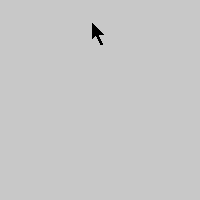# Flocking

example processing animation arraylist flocking emergence

This program takes the previous Flocking example and uses an `ArrayList` to allow the user to add `Flocker` instances by dragging the mouse.

You can technically do this using an array and the `append()` function, but using an `ArrayList` is much faster.

``````ArrayList<Flocker> flock = new ArrayList<Flocker>();

void setup() {
size(200, 200);
}

void draw() {

background(200);

for (Flocker f : flock) {
f.step();
f.draw();
}
}

void mouseDragged() {
}

class Flocker {

float x;
float y;
float speed = random(1, 3);

public Flocker(float x, float y) {
this.x = x;
this.y = y;
}

void step() {

//we need more than one Flocker for this to work
if (flock.size() > 1) {

//find the closest Flocker
float closestDistance = 100000;
Flocker closestFlocker = null;
for (Flocker f : flock) {

//make sure not to check against yourself
if (f != this) {
float distance = dist(x, y, f.x, f.y);
if (distance < closestDistance) {
closestDistance = distance;
closestFlocker = f;
}
}
}

float angleToClosest = atan2(closestFlocker.y-y, closestFlocker.x-x);

//prevent case where heading is 350 and angleToClosest is 10
angleToClosest += TWO_PI;
} else if (angleToClosest-heading > PI) {
angleToClosest -= TWO_PI;
}

//turn towards closest
} else {
}

//move in direction

//wrap around edges
if (x < 0) {
x = width;
}
if (x > width) {
x = 0;
}

if (y < 0) {
y = height;
}
if (y > height) {
y = 0;
}
}
}

void draw() {
}
}
``````Code Editor

See the Pen by Happy Coding (@KevinWorkman) on CodePen.

Watch each `Flocker` and notice that it’s just continuously trying to follow its closest neighbor. Even though each individual `Flocker` is only following simple rules, the program itself is a basic simulation of how birds flock, or how bugs swarm, or how a school of fish swims. This idea of complicated (or even beautiful) patterns emerging from simple rules is called emergence!

## Tweak Ideas

• The flocking logic here is very simple and just uses cohesion (get close to your neighbors). Better simulations also take separation (don’t get too close to your neighbors) and alignment (fly in the same direction as neighbors) into account. You could also consider the closest 5 (or 10, or…) neighbors instead of only the closest one, or you could consider all of the neighbors within some distance.
• Give each `Flocker` a random color.
• Move the call to `background()` to the `setup()` function to show trails.
• Make them run away from each other instead of chase each other.
• Make one `Flocker` “it” and have it run away from all of the other `Flockers` while they chase it- or vice-versa!